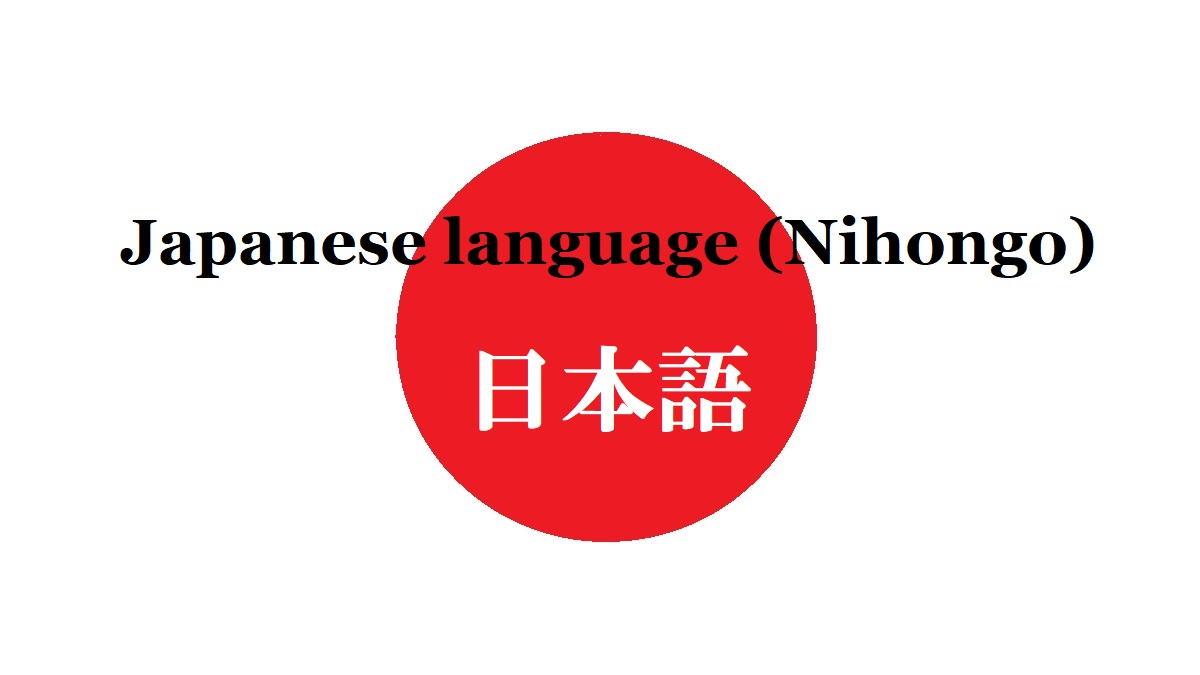HOME About Japan Language

# [Step.9] Expression of number

When you travel around Japan, it may be important to know Japanese words of number.

## Number

Basic reading of number is the following.
Some numbers have another reading in parentheses.

The reading of number in Japanese is based on decimal system.
Therefore, you read the number of each digit from the upper digits.

From 11 to 19, the reading is the following.

The reading of the ten's place is (number)+"jû".

From 21 to 99, you connect reading of the ten's place and the reading between 1 and 9.

The reading of the hundred's place is "hyaku" ().
But a few numbers are changed for making the pronunciation smooth. (I show them by changed color.)

The reading from 101 to 999 is the following.
When a digit is 0, it doesn't be read.

The reading of the thousand's place is "sen" ().
But a few numbers are changed for making the pronunciation smooth.

The reading from 1001 to 9999 is the following.
When the digit of 0, it doesn't be read.

## Large number

You have already been able to read the numbers from 0 to 9,999 in Japanese.
This means that you can read larger numbers easily.

I show a 16-digit number.

You can read the large number in each group as a 4-digit number.
It is OK to add the unit word on the upper part of the example after the 4-digit number.

“Man” () means 10,000 (ten thousand), “oku” () means 100,000,000 (one hundred million), and “chô” () means 1,000,000,000,000 (one thousand billion).

18,675
ichi-man-hassen-roppyaku-nanajû-go
5,730,412
gohyaku-nanajû-san-man-yonhyaku-jû-ni
84,652,116,383
happyaku-yonjû-roku-oku-gosen-nihyaku-jûichi-man-rokusen-sanbyaku-hachijû-san

Number is read every group of 4 digits in Japanese.
But the notation of number in Japan is every 3 digits.
It is the same as many countries in the world.

When you visit Japan, I think you use the number until the range of “man” at most.
Millionair treats the money of “oku” and Japan’s national budget treats the range of “chô”.

Probably, price is the number which you see most frequently in Japan.
Yen () is Japanese currency, and the symbol is “¥”.

The notaion is "Yen", but the pronunciation is "en" simply.

## Decimal

In Japan, period is used as decimal point.
The reading in Japanese is “ten” meaning “dot” in English.

Reading of decimal in Japanese is easy.
Each of numerical figures after a decimal point is read as a number.
0 is read as either "zero" or "rei".

When integer part is 0, it is usually read as “rei”.
And, when the numerical figure of one’s place of the integer part is 2 (ni) or 5 (go), it is usually read long like “nî” or “gô”.

## Fraction

Reading of fraction in Japanese is also easy.

(Denominator) bun no (Numerator)

Note that you must read denominator first in reverse order of notation.

English has the words such as “half” (1/2) and “quarter” (1/4).
In Japanese, there is only word “hanbun” (半分) equivalent to “half”.